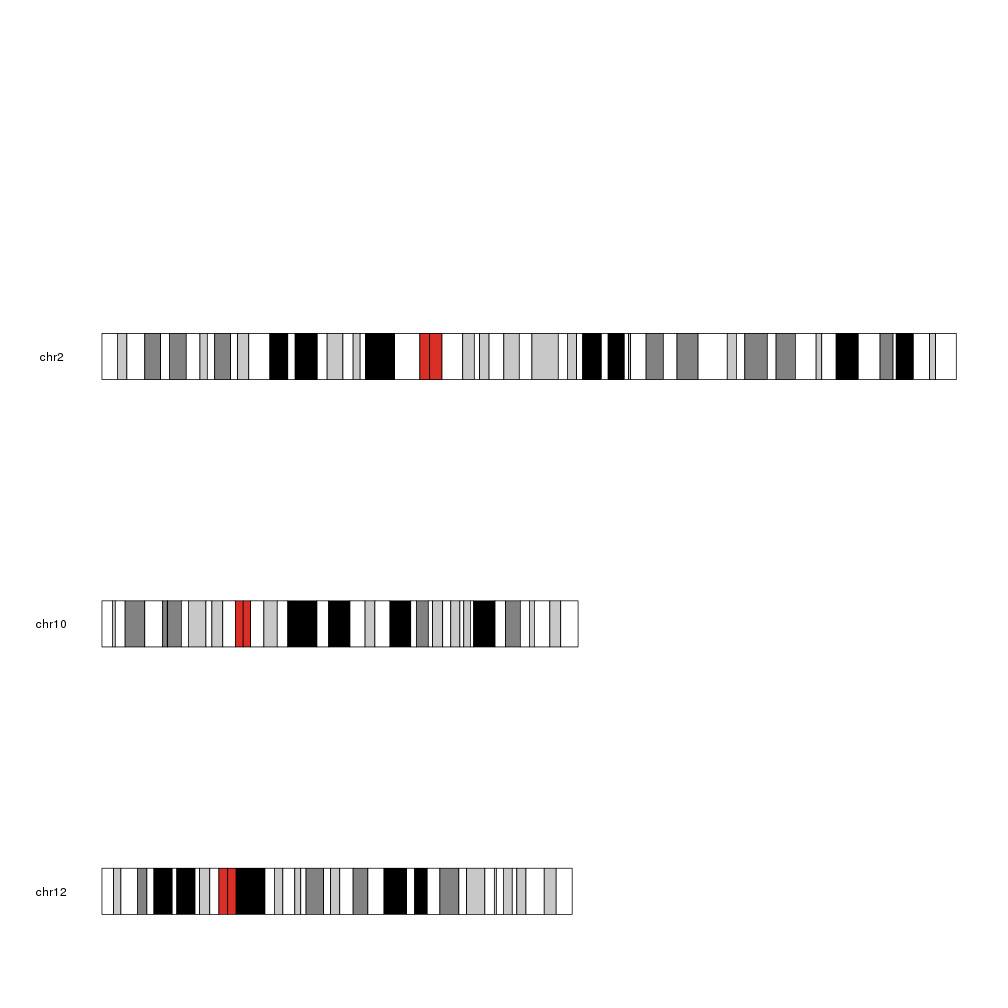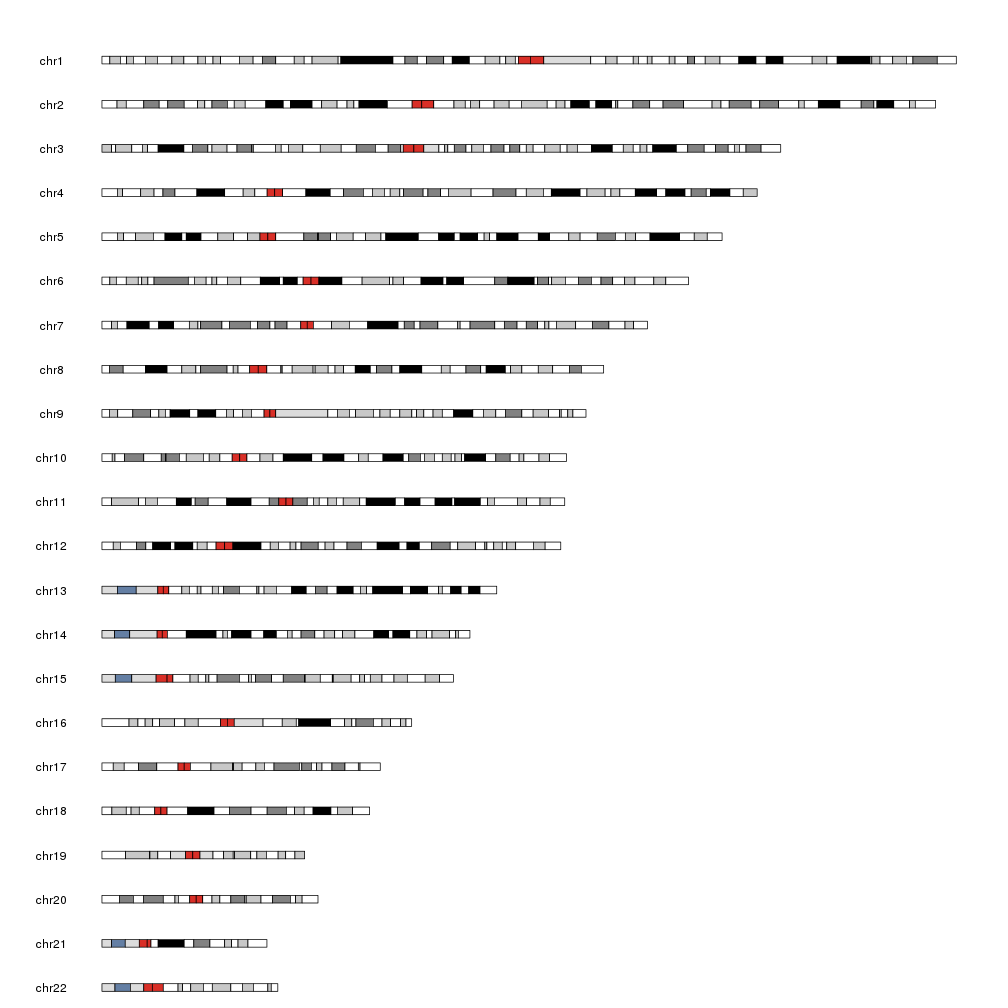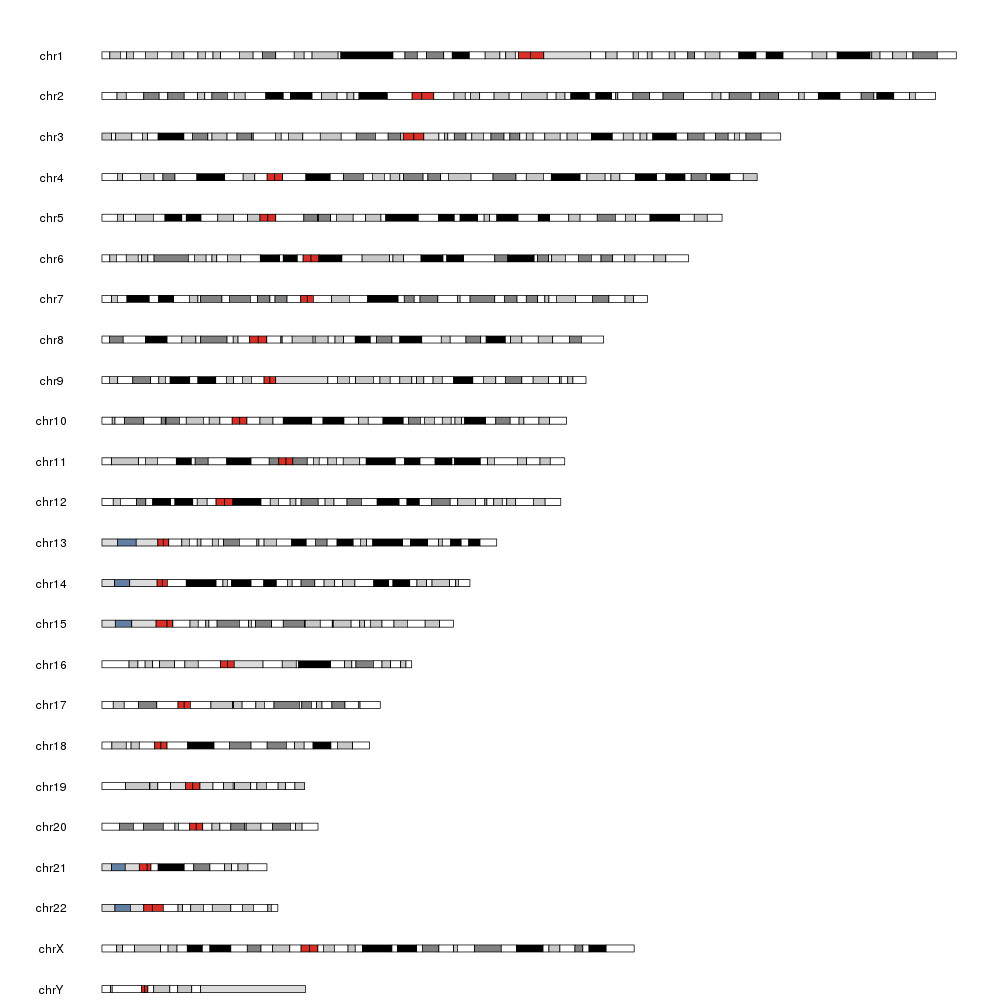Filter and Reorder Chromosomes

When plotting a karyoplot it is possible to plot only a subset of chromosomes. The selected chromosomes are specified using the chromosomes parameter.

We can specify a vector of chromosome names (note that the chromosomes will be plotted in the spcified order):

kp <- plotKaryotype(genome = "hg19", chromosomes=c("chr10", "chr12", "chr2"))Since internally plotKaryotype uses regioneR’s filterChromosomes, we can specify predefined sets of chromosomes in many genomes. By default it only plots canonical chromosomes.

For example, we can plot only autosomal chromosomes in hg19

kp <- plotKaryotype(genome = "hg19", chromosomes="autosomal")Chromosome specification can also be used to reorder the chromosomes, so for example, to plot the canonical in reverse order, we can simply specify them as:

kp <- plotKaryotype(genome = "hg19", chromosomes=rev(paste0("chr", c(1:22, "X", "Y"))))